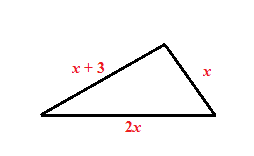### Home > AC > Chapter 8 > Lesson 8.1.3 > Problem8-27

8-27.

The perimeter of a triangle is $51$ cm. The longest side is twice the length of the shortest side. The third side is $3$ cm longer than the shortest side. How long is each side? Write an equation that represents the problem and then solve it.Write an equation for the problem.

$x+(x+3)+2x=51$

$\begin{array}{r} 4x + 3 = 51 \\ 4x = 48 \\ x = 12 \end{array}$